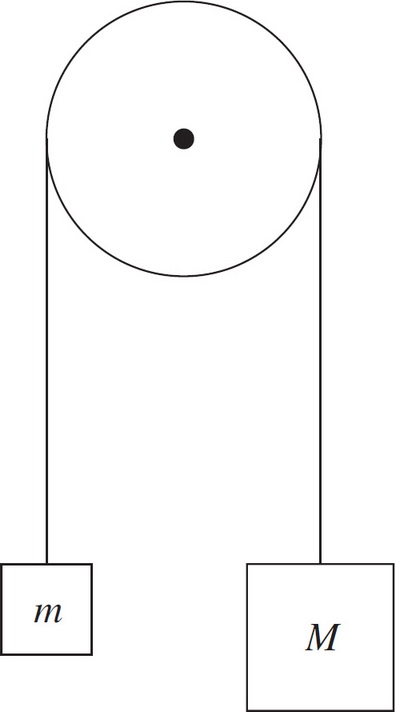# AP Physics 1: Newton's Laws Multiple-Choice Practice Questions

### Test Information10 questions18 minutes

1. A person standing on a horizontal floor feels two forces: the downward pull of gravity and the upward supporting force from the floor. These two forces

2. A person who weighs 800 N steps onto a scale that is on the floor of an elevator car. If the elevator accelerates upward at a rate of 5 m/s2, what will the scale read?

3. A frictionless inclined plane of length 20 m has a maximum vertical height of 5 m. If an object of mass 2 kg is placed on the plane, which of the following best approximates the net force it feels?

4. A 20 N block is being pushed across a horizontal table by an 18 N force. If the coefficient of kinetic friction between the block and the table is 0.4, find the acceleration of the block.

5. The coefficient of static friction between a box and a ramp is 0.5. The ramp's incline angle is 30°. If the box is placed at rest on the ramp, the box will do which of the following?6. Assuming a frictionless, massless pulley, determine the acceleration of the blocks once they are released from rest.

7. If all of the forces acting on an object balance so that the net force is zero, then

8. A block of mass m is at rest on a frictionless, horizontal table placed in a laboratory on the surface of the Earth. An identical block is at rest on a frictionless, horizontal table placed on the surface of the Moon. Let F be the net force necessary to give the Earth-bound block an acceleration of a across the table. Given that gMoon is one-sixth of gEarth, the force necessary to give the Moon-bound block the same acceleration a across the table is

9. A crate of mass 100 kg is at rest on a horizontal floor. The coefficient of static friction between the crate and the floor is 0.4, and the coefficient of kinetic friction is 0.3. A force F of magnitude 344 N is then applied to the crate, parallel to the floor. Which of the following is true?

10. Two crates are stacked on top of each other on a horizontal floor; Crate #1 is on the bottom, and Crate #2 is on the top. Both crates have the same mass. Compared to the strength of the force F1 necessary to push only Crate #1 at a constant speed across the floor, the strength of the force F2 necessary to push the stack at the same constant speed across the floor is greater than F1 because